## Filters

Sort by :
Clear All
Q

4. ABC is an isosceles triangle with and is one of its altitudes (Fig 7.34).

(i) State the three pairs of equal parts in and .
(ii) Is ? Why or why not?
(iii) Is ? Why or why not?
(iv) Is ? Why or why not?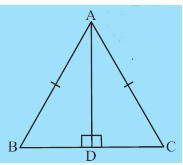i) Given in and .    ( Common side)    ii) So, by RHS Rule of congruency, we conclude   iii) Since both triangles are congruent all the corresponding parts will be equal. So,   iv) Since both triangles are congruent all the corresponding parts will be equal. So, .

3. In Fig 7.33, and are altitudes of  such that .

(i) State the three pairs of equal parts in and .
(ii) Is  ? Why or why not?
(iii) Is  ? Why or why not?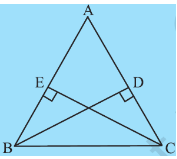i) Given,  in and .     ii) So, By RHS Rule of congruency, we conclude:   iii) Since both the triangle are congruent, all parts of one triangle are equal to their corresponding part from another triangle. So. .

2. It is to be established by RHS congruence rule that  . What additional information is needed, if it is given that

and

To prove congruency by RHS (Right angle, Hypotenuse, Side ) rule, we need hypotenuse and side equal to the corresponding hypotenus and side of different angle. So Given   ( Right angle )    ( Side ) So the third information we need is the equality of  Hypotenuse of both triangles. i.e. Hence, if this information is given then we can say, .

1.  In Fig 7.32, measures of some parts of triangles are given.By applying RHS congruence rule, state which pairs of triangles are congruent. In case of congruent triangles, write the result in symbolic form.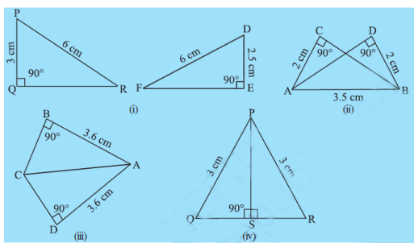i) In  and  Hence they are not congruent. ii)  In  and   ( same side ) So, by RHS congruency rule, iii) In  and   ( same side ) So, by RHS congruency rule, iv)  In  and   ( same side ) So, by RHS congruency rule,

5. In Fig 7.28, ray AZ bisects  as well as .

(i) State the three pairs of equal parts in triangles  and .
(ii) Is  Give reasons.
(iv) Is  Give reasons.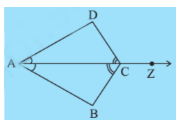i) Given in triangles  and   ( common side) ii) So, By ASA congruency criterion,triangles  and  are congruent. iii) Since  , all corresponding parts will be equal. So . iv) Since  , all corresponding parts will be equal. So

4. Given below are measurements of some parts of two triangles. Examine whether the two triangles are congruent or not, by ASA congruence rule. In the case of congruence, write it in symbolic form.

i) Given in  and  . So, by ASA congruency criterion, they are congruent to each other.i.e. . ii) Given in  and  . For congruency by ASA criterion, we need to be sure of equity of the side which is joining the two angles which are equal to their corresponding parts. Here the side QR is not given which is why we cannot conclude the congruency of both the triangles. iii) Given in  and  . For...

3. In Fig 7.27, measures of some parts are indicated. By applying ASA congruence rule, state which pairs triangles are congruent. In case of congruence, write the result in symoblic form.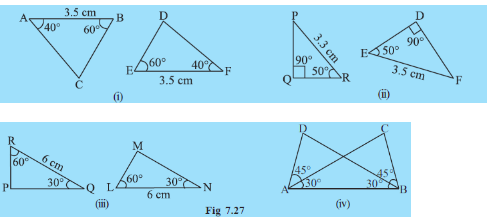i) in  and  AB = FE = 3.5 cm  So by ASA congruency rule, both triangles are congruent.i.e. ii) in  and  But, So, given triangles are not congruent. iii) in  and  RQ = LN = 6 cm  So by ASA congruency rule, both triangles are congruent.i.e. . iv) in  and  AB = BA (common side) So by ASA congruency rule, both triangles are congruent.i.e.

4. In Fig 7.25, and bisect each other at .

(i) State the three pairs of equal parts in two triangles and .

(ii) Which of the following statements are true?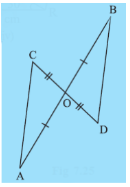i) The three pairs of equal parts in two triangles and  are: CO = DO (given) OA = OB (given )  ( As opposite angles are equal when two lines intersect.) ii) So by SAS congruency rule,  that is  Hence, option B is correct.

2. You want to establish , using the ASA congruence rule. You are given that and. What information is needed to establish the congruence? (Draw a rough figure and then try!)

As we know, in ASA congruency two angles and one side is equated to their corresponding parts. So To Prove  And The side joining these angles is   . So the information that is needed in order to prove congruency is .

1. What is the side included between the angles and N of ?

The side MN is the side which is included between the angles and N of .

3. In Fig 7.24, measures of some parts of the triangles are indicated. By applying SAS congruence rule, state the pairs of congruent triangles, if any, in each case. In case of congruent triangles, write them in symbolic form.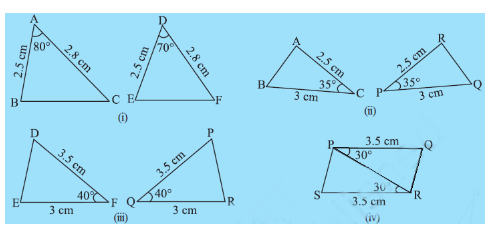i) in  and  AB = DE  AC = DF Hence, they are not congruent.   ii) In  and  AC = RP = 2.5 cm CB = PQ = 3 cm  Hence by SAS congruency rule, they are congruent. .   iii) In  and  DF= PQ = 3.5 cm FE= QR = 3 cm Hence, by SAS congruency rule, they are congruent.   iv) In  and  QP = SR = 3.5 cm PR = RP (Common side) Hence, by SAS congruency rule, they are congruent. .

2. By applying SAS congruence rule, you want to establish that  . It is given that and . What additional information is needed to establish the congruence?

To prove congruency by SAS rule, we need to equate two corresponding sides and one corresponding angle, so in   proving  we need, And . Hence the extra information we need is .

1. Which angle is included between the sides   and of  ?

Since both the sides    and intersects at E,   is included between the sides   and of  .

1.  ABC is an isosceles triangle with  (Fig 7.17). Take a trace-copy of  and also name it as
(i) State the three pairs of equal parts in .
(ii) Is ? Why or why not?
(iii) Is  ? Why or why not?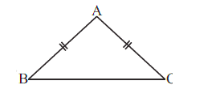Here, in .    i)the three pairs of equal parts in  are AB = AC  BC = CB  AC = AB  ii) Hence By SSS Congruency rule, they both are congruent. iii) Yes,  because  are congruent and by equating the corresponding parts of the triangles we get, .

3. In Fig 7.16,   and . Which of the following statements is meaningfully written?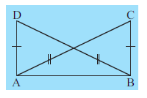Given,   and . AB = AB ( common side ) So By SSS congruency rule,  . So this statement is meaningfully written as all given criterions are satisfied in this.

2.  In Fig 7.15,  and D is the mid-point of  .

(i) State the three pairs of equal parts in  and
(ii) Is  Give reasons.
(iii) Is  Why?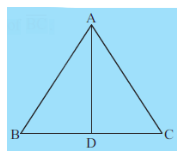Here in   and i) Three pair of equal parts are: AD = AD ( common side )  BD = CD ( as d is the mid point of BC) AB = AC (given in the question) ii) Now,   by SSS Congruency rule, iii) As both triangles are congruent to each other we can compare them and say .

1. In Fig 7.14, lengths of the sides of the triangles are indicated. By applying the SSS congruence rule, state which pairs of triangles are congruent. In case of congruent triangles, write the result in symbolic form: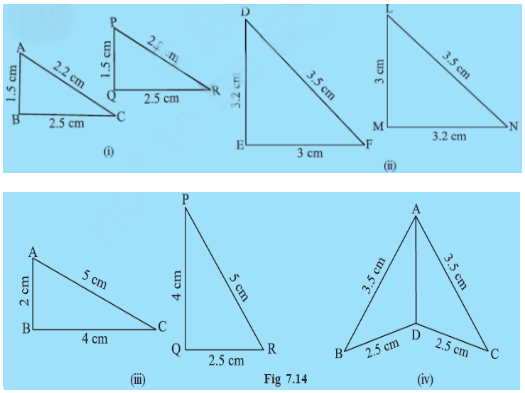i) Since  AB = PQ BC = QR CA = PR So, by SSS congruency rule both triangles are congruent to each other.   ii) Since, ED = MN DF = NL  FE = LM So, by SSS congruency rule both triangles are congruent to each other. .   iii) Since  AC = PR  BC = QR But So the given triangles are not congruent.   iv) Since, AD = AD  AB = AC  BD = CD  So, By SSS Congruency rule, they both are congruent to each other. .

1.   When two triangles, say ABC and PQR are given, there are, in all, six possible matchings or correspondences. Two of them are:

and

Find the other four correspondences by using two cutouts of triangles. Will all these correspondences lead to congruence? Think about it.

the other four correspondences by using two cutouts of triangles are :
Exams
Articles
Questions